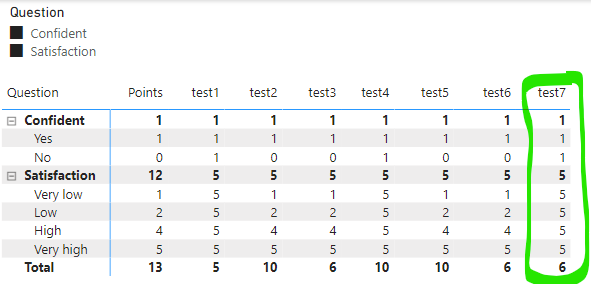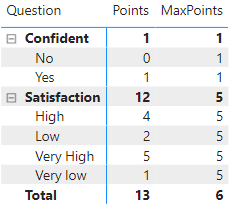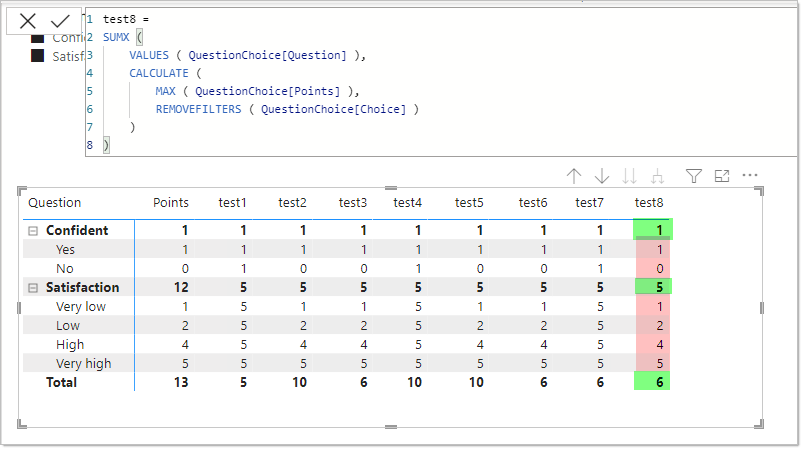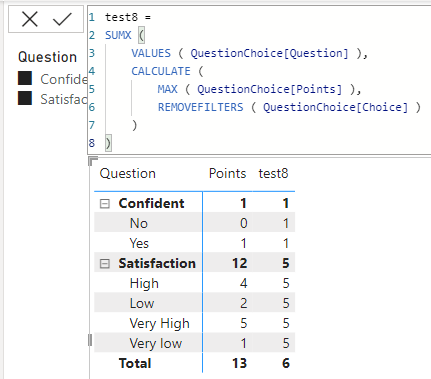cancel
Showing results for
Did you mean:

Fabric is Generally Available. Browse Fabric Presentations. Work towards your Fabric certification with the Cloud Skills Challenge.## Level of detail SUM of MAXs

Hi,

I solved my problem myself but I'm unhappy with the solution and I would love to hear from an expert to figure out what is it that always seems to elude me about how DAX really works.

I simplified it with a model containing a single small table.  It a dimension table about a survey.  One question about your level of satisfaction and a second question asking if you are confident or not.  There are points linked to each possible answer.The result I'm looking for can be seen below in column test7.  For every detailed row (Choice level), I want to see the maximum points you can get for this question.  Then at Question level I want to see the maximum points you can get for this question.  Finally, for the grand total, I want to see the sum of the max points of every selected questions (notice the slicer).test1 : Choice and Question levels are correct but the grand total shows the max point of a single question.  I need the sum of max.

``CALCULATE ( MAX ( QuestionChoice[Points] ), REMOVEFILTERS(QuestionChoice), VALUES(  QuestionChoice[Question]) )``

test2 : I thought this would give me Sum of Max for the grand total but it seems like the Summarized calculation returns 5 points even for the question where the max in supporsed to be 1.

``````VAR _t = ADDCOLUMNS(
SUMMARIZE( QuestionChoice, QuestionChoice[Question]),
"maxPoints", MAX( QuestionChoice[Points] )
)
RETURN SUMX( _t, [maxPoints])``````

test3 : trying to add a Calculate() to for context evaluation.  It works... but now it's wrong at the lower Choice level.

``````VAR _t = ADDCOLUMNS(
SUMMARIZE( QuestionChoice, QuestionChoice[Question]),
"maxPoints",CALCULATE( MAX( QuestionChoice[Points] ) )
)
RETURN SUMX( _t, [maxPoints])``````

test4 : I'm thinking ok then, let's remove all filters then apply one at the Question Level.  Yay, it fixed Choice level row... Booo!  the grand total is wrong again.  Here I really don't get why!? I was certain by doing both Summarized on QuestionChoice[Question] and Calculate filtered with VALUES(QuestionChoice[Question]), there was no way the maxPoint for the Yes/No question would be equal to 5.  But it seems the result is 5 + 5 = 10.

``````VAR _t = ADDCOLUMNS(
SUMMARIZE( QuestionChoice, QuestionChoice[Question]),
"maxPoints", CALCULATE( MAX (QuestionChoice[Points] ), REMOVEFILTERS(QuestionChoice), VALUES(QuestionChoice[Question]) )
)
RETURN SUMX( _t, [maxPoints])``````

test5 : Maybe I removed to many filters?... Let's try remoing filter only in Choice.  Well, that didn't work.  Everything is wrong now 😞

``````VAR _t = ADDCOLUMNS(
SUMMARIZE( QuestionChoice, QuestionChoice[Question]),
"maxPoints", CALCULATE( MAX( QuestionChoice[Points] ), REMOVEFILTERS(QuestionChoice[Choice]), VALUES(QuestionChoice[Question]) )
)
RETURN SUMX( _t, [maxPoints])``````

test6 : Ok, since test1 formula gives the correct detail and sous-total results; and test3 gives the correct grand total, let's call the right formula for the right scenario.  WTH ?!?! this doesn't work!  why?

``````VAR _test1 = CALCULATE( MAX (QuestionChoice[Points] ), REMOVEFILTERS(QuestionChoice[Choice]), VALUES(QuestionChoice[Question]) )
SUMMARIZE( QuestionChoice, QuestionChoice[Question]),
"maxPoints", CALCULATE( MAX( QuestionChoice[Points] ) )
)
RETURN  IF( HASONEVALUE( QuestionChoice[Question]), _test1,  SUMX( _t, [maxPoints]) )``````

test7 : Hmmm, what if I call the measures instead of using VARiables?  Eureka!  that works!  Finally!

``IF( HASONEVALUE( QuestionChoice[Question]), [test1],  [test3] )``

Questions :

1. Is there a better way to do this?  For instance, is it possible to do it with 1 measure instead of 3?
2. Why does test4 not working?
3. Why does test6 and test7  not equal?

Thanks

--mo

1 ACCEPTED SOLUTIONSuper User

Ah, I should have figured this out from the model screenshot...

You have an extra [Sort] column that is throwing things off.  I removed the [Choice] filter but [Sort] was still part of the filter context. I recommend using ALLEXCEPT instead, which allows us to specify the context we want to keep rather than what to remove.

``````test9 =
SUMX (
VALUES ( QuestionChoice[Question] ),
CALCULATE (
MAX ( QuestionChoice[Points] ),
ALLEXCEPT ( QuestionChoice, QuestionChoice[Question] )
)
)``````
11 REPLIES 11Super User

You're so close!

Here's how I would write it:

``````MaxPoints =
SUMX (
VALUES ( QuestionChoice[Question] ),
CALCULATE (
MAX ( QuestionChoice[Points] ),
REMOVEFILTERS ( QuestionChoice[Choice] )
)
)``````Hi @AlexisOlson ,

I copy-paste the formula you suggested but I don't get the same results.  The Choice-level results are not as expected (highlighted in pink in the image below)

I'm confused!

Do you have any idea what is happening?Super User

Odd. It works for me. Maybe you have some table relationships I'm unaware of?Hi,

I would love to share the document with you but I don't see any option to attach a document and I dont think my organisation would appreciate if I post on a public forum a shared link to a document located on our private SharePoint server 😞

The pbix file doesn't have any sensitive data though.  Do you know if Microsoft offers a public cloud space where we can share files related to topics in this community forum?

in the meantime, here's a screenshot of my very simple data model.Super User

You can publicly share non-sensitive files on any number of cloud hosts like OneDrive/SharePoint/GoogleDrive/Dropbox etc. or use a more anonymous service like the ones mentioned here.Super User

Ah, I should have figured this out from the model screenshot...

You have an extra [Sort] column that is throwing things off.  I removed the [Choice] filter but [Sort] was still part of the filter context. I recommend using ALLEXCEPT instead, which allows us to specify the context we want to keep rather than what to remove.

``````test9 =
SUMX (
VALUES ( QuestionChoice[Question] ),
CALCULATE (
MAX ( QuestionChoice[Points] ),
ALLEXCEPT ( QuestionChoice, QuestionChoice[Question] )
)
)``````My fault!  I actually cut out the sort column in the first screenshot I took just because I wanted to "keep it simple".

I actually used to use ALLEXCEPT until I read this article (Using ALLEXCEPT versus ALL and VALUES - SQLBI) that suggests using REMOVEFILTERS + VALUES is a better way.  I respect Alberto Ferrari’s work a lot! … but I guess I should’ve been more aware of my particular scenario.

I'm SO frustrated with this Power BI + DAX ! I have the skills to edit my PBIX files in VSCode but I don’t seem to be able to write the simplest DAX expression ☹ As a computer engineer, I’m usually not satisfy when something works; I need to understand why it's working.  But with DAX I mostly have to guess, with many trialssss and errorssss, until “pop!”, some magic trick makes it work.

In my 20-year career in BI I’ve developed in T-SQL, C++, C#, Java, Python, R, I wrote shell scripts, I modeled normalized databases, I know Kimbal vs Inmon methodologies, I designed start models and data services,  I used tool like Crystal Report, Business Objects, Cognos, QlickView, I’m a certified Tableau professional… yet the last 2 years, working with Power BI and especially DAX as been the challenge of my career!

So far, my training as a computer engineer had been enough to be able to learn all sort of new things by myself… this time I guess I will have to be humble and accept that I need to go back to school, at least for a bit, and get down to those fundamental concepts like “context filters”, “context transition” and “iterator functions”.

Anyway, sorry for the editorial! Lol

And thank you so much for taking the time to answer my question! 😊

--moSuper User

DAX does indeed have hidden depths. Thanks for the link. I'm not sure I'd read that one (at least not recently) and have learned some new things to keep in mind. Definitely an alternative pattern worth remembering.

Should we consider the following as the solution?

``````test10 =
SUMX (
VALUES ( QuestionChoice[Question] ),
CALCULATE (
MAX ( QuestionChoice[Points] ),
REMOVEFILTERS ( QuestionChoice),
VALUES ( QuestionChoice[Question] )
)
)``````

Edit: Nevermind. It looks like you did accept a solution.Yes, I accepted your test9 answer.  thanks again.

But you're right.  Based on considerations mentionned in Alberto Ferrari's article, I would think your test10 would be a better syntaxe.  But it doesn't work for the grand total row.  It's similar to what I tried in test4... and I have absolutely no idea why neither test4 nor test10 work while test9 does.  I'm baffled !``````//test4 =
SUMMARIZE( QuestionChoice, QuestionChoice[Question]),
"maxPoints",
CALCULATE(
MAX (QuestionChoice[Points] ),
REMOVEFILTERS(QuestionChoice),
VALUES(QuestionChoice[Question]) )
)
RETURN SUMX( _t, [maxPoints]);

//test9 =
SUMX (
VALUES ( QuestionChoice[Question] ),
CALCULATE (
MAX ( QuestionChoice[Points] ),
ALLEXCEPT ( QuestionChoice, QuestionChoice[Question] )
)
);

//test10 =
SUMX (
VALUES ( QuestionChoice[Question] ),
CALCULATE (
MAX ( QuestionChoice[Points] ),
REMOVEFILTERS ( QuestionChoice),
VALUES ( QuestionChoice[Question] )
)
);``````Super User

In test4 and test10, the VALUES inside CALCULATE is being evaluated in the original filter context rather than the row context of the SUMX iterator since the context transition doesn't automatically apply to the filter argument(s) of CALCULATE.

Here are some alternatives that address this:

``````test11 =
SUMX (
VALUES ( QuestionChoice[Question] ),
CALCULATE (
MAX ( QuestionChoice[Points] ),
REMOVEFILTERS ( QuestionChoice ),
CALCULATETABLE ( VALUES ( QuestionChoice[Question] ) )
)
)

test12 =
SUMX (
VALUES ( QuestionChoice[Question] ),
CALCULATE (
CALCULATE (
MAX ( QuestionChoice[Points] ),
REMOVEFILTERS ( QuestionChoice ),
VALUES ( QuestionChoice[Question] )
)
)
)

test13 =
SUMX (
VALUES ( QuestionChoice[Question] ),
CALCULATE (
MAX ( QuestionChoice[Points] ),
REMOVEFILTERS ( QuestionChoice ),
QuestionChoice[Question] = EARLIER ( QuestionChoice[Question] )
)
)``````Announcements#### Power BI Monthly Update - November 2023

Check out the November 2023 Power BI update to learn about new features.#### Fabric Community News unified experience

Read the latest Fabric Community announcements, including updates on Power BI, Synapse, Data Factory and Data Activator.#### The largest Power BI and Fabric virtual conference

130+ sessions, 130+ speakers, Product managers, MVPs, and experts. All about Power BI and Fabric. Attend online or watch the recordings.Top Solution Authors
Top Kudoed Authors
Users online (2,971)